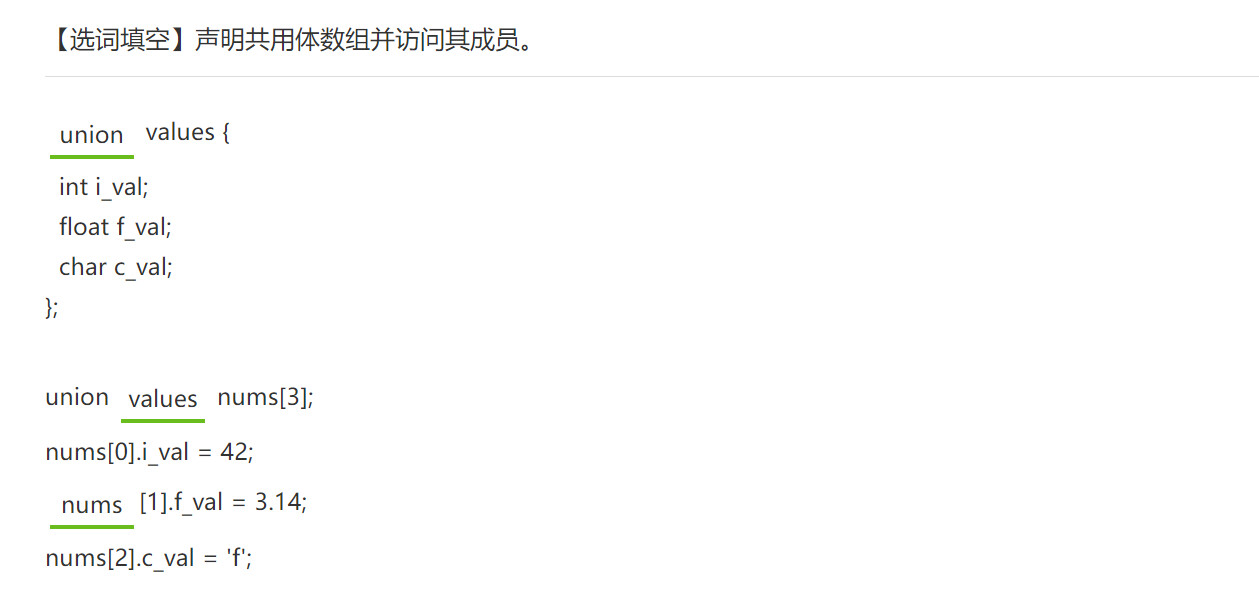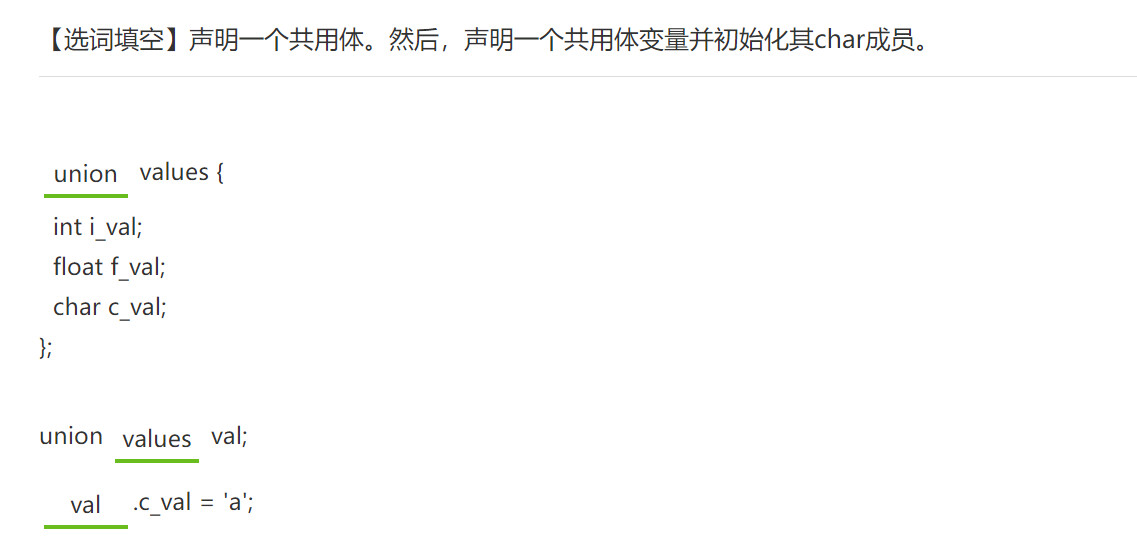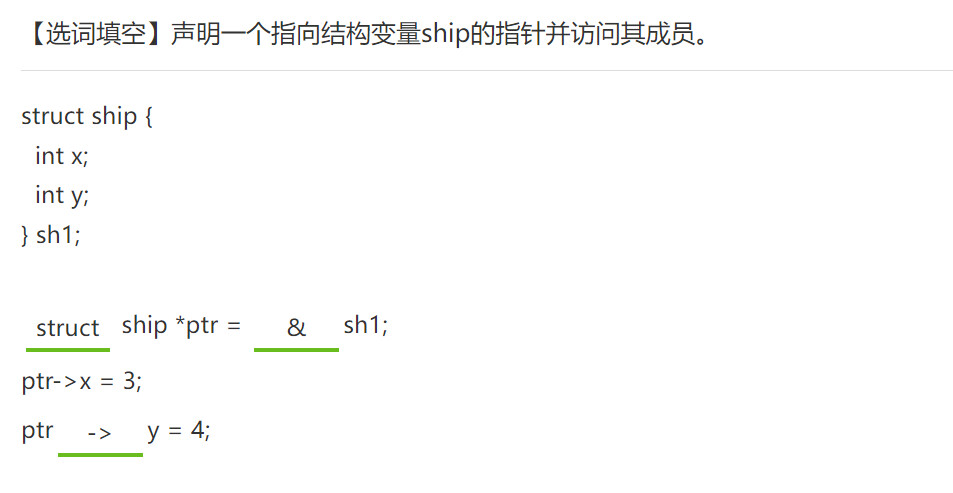Note

# C语言超级无敌速成

2022年08月11日 Thursday · 56 min read

## 使用内存

C被设计为一种低级语言，可以轻松访问内存位置并执行与内存相关的操作。

int num;
printf("Enter a number: ");

scanf("%d", &num);

printf("%d", num);


＆num是变量num的地址。

void test(int k);

int main() {
int i = 0;

printf("The address of i is %x\n", &i);
test(i);
printf("The address of i is %x\n", &i);
test(i);

return 0;
}

void test(int k) {
printf("The address of k is %x\n", &k);
}


The address of i is 846dd754
The address of k is 846dd758
The address of i is 846dd754
The address of k is 846dd758


## 什么是指针？

指针数据类型 *标识符


int j = 63;
int *p = NULL;
p = &j;

printf("The address of j is %x\n", &j);
printf("p contains address %x\n", p);
printf("The value of j is %d\n", j);
printf("p is pointing to the value %d\n", *p);


The address of j is ff3652cc
p contains address ff3652cc
The value of j is 63
p is pointing to the value 63


int x = 12;
int *p = NULL
int **ptr = NULL;
p = &x;
ptr = &p;

## 表达式中的指针

int x = 5;
int y;
int *p = NULL;
p = &x;

y = *p + 2; /* y 被赋予 7 */
y += *p;     /* y 被赋予 12 */
*p = y;       /* x 被赋予 12 */
(*p)++;      /* x 增加到 13 */

printf("p指向值 %d\n", *p);


p指向值 13


## 指针和数组

int *p, a;
p=a;


int *p, a;
p = &a;


int a = {22, 33, 44, 55, 66};
int *ptr = NULL;
int i;

ptr = a;
for (i = 0; i < 5; i++) {
printf("%d ", *(ptr + i));
}


## 更多地址算法

int a = {22, 33, 44, 55, 66};
int *ptr = NULL;

ptr = a;  /* point to the first array element */
printf("%d  %x\n", *ptr, ptr);  /* 22 */
ptr++;
printf("%d  %x\n", *ptr, ptr);  /* 33 */
ptr += 3;
printf("%d  %x\n", *ptr, ptr);  /* 66 */
ptr--;
printf("%d  %x\n", *ptr, ptr);  /* 55 */
ptr -= 2;
printf("%d  %x\n", *ptr, ptr);  /* 33 */


22 febd4760
33 febd4764
66 febd4770
55 febd476c
33 febd4764


## 指针与函数

void swap (int *num1, int *num2);

int main() {
int x = 25;
int y = 100;

printf("x is %d, y is %d\n", x, y);
swap(&x, &y);
printf("x is %d, y is %d\n", x, y);

return 0;
}

void swap (int *num1, int *num2) {
int temp;

temp = *num1;
*num1 = *num2;
*num2 = temp;
}


## 具有数组参数的函数

int add_up (int *a, int num_elements);

int main() {
int orders = {100, 220, 37, 16, 98};

printf("Total orders is %d\n", add_up(orders, 5));

return 0;
}

int add_up (int *a, int num_elements) {
int total = 0;
int k;

for (k = 0; k < num_elements; k++) {
total += a[k];
}

return (total);
}


Total orders is 471


## 返回数组的函数

int * get_evens();

int main() {
int *a;
int k;

a = get_evens(); /* get first 5 even numbers */
for (k = 0; k < 5; k++)
printf("%d\n", a[k]);

return 0;
}

int * get_evens() {
static int nums;
int k;
int even = 0;

for (k = 0; k < 5; k++) {
nums[k] = even += 2;
}

return (nums);
}


#include<stdio.h>
int * test() {
static int x;
for(int i=0;i<4;i++){
x[i] = i%2;
}
return x;
}

int main() {
int * arr = test();
printf("%d", *(arr+3));
}

输出结果为 1


## 字符串

C中的字符串是一个以NULL字符'\0'结尾的字符数组。

char str_name[str_len] = "string";


char str1 = "hello";
char str2[ ] = "world";  /* size 6 */


char str3 = {'h', 'e', 'l', 'l', 'o', '\0'};
char str4[ ] = {'h', 'e', 'l', 'l', 'o', '\0'}; /* size 6 */


strlen() -获取字符串的长度

strcat()-合并两个字符串

strcpy()-将一个字符串复制到另一个

strlwr()-将字符串转换为小写

trupr()-将字符串转换为大写

strrev()-反向字符串

strcmp()-比较两个字符串

## 字符串输入

char first_name;
int age;
printf("Enter your first name and age: \n");
scanf("%s %d", first_name, &age);


scanf()到达空格时停止读取输入。

char full_name;
printf("Enter your full name: ");
gets(full_name);


gets()的更安全替代方法是fgets()，它最多读取指定数量的字符。

char full_name;
printf("Enter your full name: ");
fgets(full_name, 50, stdin);


fgets() 参数是字符串名称，要读取的字符数以及指向要从中读取字符串的指针。 stdin表示从标准输入（即键盘）中读取。

get 和 fgets 之间的另一个区别是换行符由fgets存储。

fgets() 仅从stdin读取n-1个字符，因为必须有用于'\0'的空间。

## 字符串输出

fputs 函数

fputs 函数的作用是想指定的文件写入一个字符串，其中字符串可以是字符串常量，也可以是字符组名、指针或变量。

fputs 一般形式如下：

fputs(字符串, 文件指针)


#include <stdio.h>
int main()
{
FILE *wj=NULL;
wj=fopen("E:\\文件夹1\\生成.txt","w+")
char city;
printf("Enter your favorite city: ");
gets(city);
//fgets(要写入的数组，大小，要读取的文件)//一般用法
// fgets(city, 40, stdin);//从stdin输入中读取40位写入city数组中 此用法与gets（city）同义
//超过40位以外的不读取！！
fputs(city, wj);//将city里的数据写入到wj
puts(city);//在终端显示city里面的数据
// fputs(city,stdout);//与puts相同
printf(" is a fun city.");

return 0;
}


puts() 函数仅接受字符串参数，也可以用于显示输出。

#include <stdio.h>
int main()
{
char city;
printf("Enter your favorite city: ");
gets(city);
// Note: for safety, use
// fgets(city, 40, stdin);

puts(city);

return 0;
}


## sprintf 和 sscanf 函数

#include <stdio.h>
int main()
{
char info;
char dept[ ] = "HR";
int emp = 75;
sprintf(info, "The %s dept has %d employees.", dept, emp);
printf("%s\n", info);

return 0;
}


The HR dept has 75 employees.


#include <stdio.h>
int main()
{
char info[ ] = "Snoqualmie WA 13190";
char city;
char state;
int population;
sscanf(info, "%s %s %d", city, state, &population);
printf("%d people live in %s, %s.", population, city, state);

return 0;
}


13190 people live in Snoqualmie, WA.


## string.h库（字符串函数）

string.h库包含许多字符串函数。

• strlen(str) 返回存储在str中的字符串的长度，不包括NULL字符。

• strcat(str1, str2) 将str2追加（连接）到str1的末尾，并返回指向str1的指针。

• strcpy(str1, str2) 将str2复制(覆盖)到str1。此功能对于为字符串分配新值很有用。

• strncat(str1, str2, n) 将str2的前n个字符追加（连接）到str1的末尾，并返回指向str1的指针。

• strncpy(str1, str2, n) 将str2的前n个字符复制(覆盖)到str1。

• strcmp(str1, str2) 当str1等于str2时返回0，在 str1 <str2 时返回小于0，在 str1> str2 时返回大于0。

• strncmp(str1, str2, n) 当str1的前n个字符等于str2的前n个字符时，返回0；当str1 <str2时，小于0；当str1> str2时，大于0。

• strchr(str1, c) 返回指向str1中首次出现的char c的指针，如果找不到字符，则返回NULL。

• strrchr(str1,c) 反向搜索str1并返回一个指向char c在str1中位置的指针；如果找不到字符，则返回NULL。

• strstr(str1,str2) 返回指向str1中首次出现的str2的指针，如果未找到str2，则返回NULL。

#include <stdio.h>
#include <string.h>

int main()
{
char s1[ ] = "The grey fox";
char s2[ ] = " jumped.";

strcat(s1, s2);
printf("%s\n", s1);
printf("Length of s1 is %d\n", strlen(s1));
strcpy(s1, s2);
printf("s1 is now %s \n", s1);

return 0;
}


The grey fox jumped.
Length of s1 is 20
s1 is now  jumped.


## 将字符串转换为数字

stdio.h库包含以下用于将字符串转换为数字的函数：

• int atoi(str) 代表ASCII转成整数。将str转换为等效的int值。如果第一个字符不是数字或未遇到任何数字，则返回0。

• double atof(str)表示ASCII转成浮动。将str转换为等效的double值。如果第一个字符不是数字或未遇到数字，则返回0.0。

• long int atol(str) 表示ASCII转成long int。将str转换为等效的长整数值。如果第一个字符不是数字或未遇到任何数字，则返回0。

#include <stdio.h>
int main()
{
char input;
int num;

printf("Enter a number: ");
gets(input);
num = atoi(input);

return 0;
}


## 字符串数组

char trip = {
"suitcase",
"passport",
"ticket"
};


char *trip[ ] = {
"suitcase",因为每个元素的长度都可以变化，所以与二维网格结构相比，字符串指针数组的结构更加参差不齐。
"passport",
"ticket"
};

printf("Please bring the following:\n");
for (int i = 0; i < 3; i++) {
printf("%s\n", trip[ i ]);
}


## 函数指针

return_type (*函数名)(参数)


(*函数名) 周围的括号很重要。没有括号，编译器会认为函数在返回指针。

#include <stdio.h>
void say_hello(int num_times); /* function */

int main() {
void (*funptr)(int);  /* function pointer */
funptr = say_hello;  /* 看注意 */
funptr(3);  /* function call */

return 0;
}

void say_hello(int num_times) {
int k;
for (k = 0; k < num_times; k++)
printf("Hello\n");
}


## 函数指针数组

#include <stdio.h>

int add(int num1, int num2);
int subtract(int num1, int num2);
int multiply(int num1, int num2);
int divide(int num1, int num2);

int main()
{
int x, y, choice, result;
int (*op)(int, int);

op = subtract;
op = multiply;
op = divide;
printf("Enter two integers: ");
scanf("%d%d", &x, &y);
printf("Enter 0 to add, 1 to subtract, 2 to multiply, or 3 to divide: ");
scanf("%d", &choice);
result = op[choice](x, y);
printf("%d", result);

return 0;
}

int add(int x, int y) {
return(x + y);
}

int subtract(int x, int y) {
return(x - y);
}

int multiply(int x, int y) {
return(x * y);
}

int divide(int x, int y) {
if (y != 0)
return (x / y);
else
return 0;
}


int (*op)(int, int); 语句声明函数指针的数组。每个数组元素必须具有相同的参数和返回类型。

## 空指针

void *ptr;


int x = 33;
float y = 12.4;
char c = 'a';
void *ptr;

ptr = &x;
printf("void ptr points to %d\n", *((int *)ptr));
ptr = &y;
printf("void ptr points to %f\n", *((float *)ptr));
ptr = &c;
printf("void ptr points to %c", *((char *)ptr));

• *(int )ptr是表示把ptr强制转换成一个int型的指针。

• **(int )ptr就是取ptr指向的内容的意思，跟*ptr的那个*作用一样

• **void指针可以保存各种其它指针类型。**大多数时候它被用来存储数据结构。

## 使用空指针的函数

void * square (const void *);


#include <stdio.h>

void* square (const void* num);

int main() {
int x, sq_int;
x = 6;
sq_int = square(&x);
printf("%d squared is %d\n", x, sq_int);

return 0;
}

void* square (const void *num) {
int result;
result = (*(int *)num) * (*(int *)num);
return result;
}


square 函数需要 int 类型，这就是为什么将num void指针强制转换为int的原因。

## 函数指针作为参数

stdlib.h头文件中的 qsort() 函数使用此技术。

quicksort是一种广泛用于对数组进行排序的算法。

void qsort(void *base, size_t nitems, size_t size, int (*compar)(const void *, const void*))


qsort声明解释：

base 指向要排序的数组的第一个元素的指针。。

nitems 由 base 指向的数组中元素的个数。

size 数组中每个元素的大小，以字节为单位。

compar 用来比较两个元素的函数，即函数指针（回调函数）

compar参数

compar参数指向一个比较两个元素的函数。比较函数的原型应该像下面这样。注意两个形参必须是const void *型，同时在调用compar 函数（compar实质为函数指针，这里称它所指向的函数也为compar）时，传入的实参也必须转换成const void *型。在compar函数内部会将const void *型转换成实际类型。

​ int compar(const void *p1, const void *p2);

​ 如果compar返回值小于0（< 0），那么p1所指向元素会被排在p2所指向元素的左面；

#include <stdio.h>
#include <stdlib.h>

int compare (const void *, const void *);

int main() {
int arr = {52, 23, 56, 19, 4};
int num, width, i;

num = sizeof(arr)/sizeof(arr);
width = sizeof(arr);
qsort((void *)arr, num, width, compare);
for (i = 0; i < 5; i++)
printf("%d ", arr[ i ]);

return 0;
}

int compare (const void *elem1, const void *elem2) {
if ((*(int *)elem1) == (*(int *)elem2))
return 0;
else if ((*(int *)elem1) < (*(int *)elem2))
return -1;
else
return 1;
}


## 结构体

“结构体”是一种构造类型，它是由若干“成员”组成的，其中的每一个成员可以是一个基本数据类型或者又是一个构造类型。既然结构体是一种新的类型，就需要先对其进行构造，这里这种操作称为声明一个结构体。

struct 结构体名
{
成员列表
}


struct course {
int id;
char title;
float hours;
};


## 结构体变量的定义

struct student {
int age;
char name;
};

/* declare two variables */
struct student s1;
struct student s2;


## 声明结构体变量

struct student s1 = {19, 9, "John"};
struct student s2 = {22, 10, "Batman"};


struct student s1;
s1 = (struct student) {19, 9, "John"};


struct student s1
= { .grade = 9, .age = 19, .name = "John"};


## 结构体变量的引用

结构体变量.成员名


s1.age = 19;


struct student s1 = {19, 9, "Jason"};
struct student s2;
//....
s2 = s1;


#include <stdio.h>
#include <string.h>

struct course {
int id;
char title;
float hours;
};

int main() {
struct course cs1 = {341279, "Intro to C++", 12.5};
struct course cs2;

/* initialize cs2 */
cs2.id = 341281;
cs2.hours = 14.25;

/* display course info */
printf("%d\t%s\t%4.2f\n", cs1.id, cs1.title, cs1.hours);
printf("%d\t%s\t%4.2f\n", cs2.id, cs2.title, cs2.hours);

return 0;
}


## 使用typedef

typedef关键字 创建一个类型定义，该定义可简化代码并使程序更易于阅读。

typedef 通常与结构一起使用，因为它消除了在声明变量时使用关键字struct的需要。

typedef struct {
int id;
char title;
float hours;
} course;

course cs1;
course cs2;


## 结构与结构

typedef struct {
int x;
int y;
} point;

typedef struct {
point center;
} circle;


circle c = {4.5, {1, 3}};
printf("%3.1f %d,%d", c.radius, c.center.x, c.center.y);
/* 4.5  1,3 */


## 指向结构的指针

struct myStruct *struct_ptr;


struct_ptr = &struct_var;


struct_ptr -> struct_mem;


struct student{
char name;
int number;
int age;
};

// Struct pointer as a function parameter
void showStudentData(struct student *st) {
printf("\nStudent:\n");
printf("Name: %s\n", st->name);
printf("Number: %d\n", st->number);
printf("Age: %d\n", st->age);
}

struct student st1 = {"Krishna", 5, 21};
showStudentData(&st1);


-> 运算符允许通过指针访问结构的成员。

(*st).age与st->age相同。

## 结构作为函数参数

#include <stdio.h>
#include <string.h>

typedef struct {
int id;
char title;
float hours;
} course;

void update_course(course *class);
void display_course(course class);

int main() {
course cs2;
update_course(&cs2);
display_course(cs2);
return 0;
}

void update_course(course *class) {
strcpy(class->title, "C++ Fundamentals");
class->id = 111;
class->hours = 12.30;
}

void display_course(course class) {
printf("%d\t%s\t%3.2f\n", class.id, class.title, class.hours);
}


## 结构数组

#include <stdio.h>

typedef struct {
int h;
int w;
int l;
} box;

int main() {
box boxes = {{2, 6, 8}, {4, 6, 6}, {2, 6, 9}};
int k, volume;

for (k = 0; k < 3; k++) {
volume = boxes[k].h*boxes[k].w*boxes[k].l;
printf("box %d volume %d\n", k, volume);
}
return 0;
}


## 共用体

• 共用体和结构体的区别在于：结构体定义了一个由多个数据成员组成的特殊类型，而共用体定义了一块为所有数据成员共享的内存。

• 共用体也称为联合体，它使几种不同类型的变量存放到同一段内存单元中。所以共用体在同一时刻只能有一个值，它属于某一个数据成员。由于所有成员位于同一块内存，因此共用体的大小就等于最大成员的大小。

union 共用体名
{
成员列表
}变量列表;


union val {
int int_num;
float fl_num;
char str;
};


union val u1;
union val u2;
u2 = u1;


## 访问共用体成员

共用体变量.成员名


union val {
int int_num;
float fl_num;
char str;
};

union val test;

test.int_num = 123;
test.fl_num = 98.76;
strcpy(test.str, "hello");

printf("%d\n", test.int_num);
printf("%f\n", test.fl_num);
printf("%s\n", test.str);


1819043176
1143141483620823940762435584.000000
hello


## 结构体内共用体

typedef struct {
char make;
int model_year;
int id_type; /* 0 for id_num, 1 for VIN */
union {
int id_num;
char VIN;
} id;
} vehicle;

vehicle car1;
strcpy(car1.make, "Ford");
car1.model_year = 2017;
car1.id_type = 0;
car1.id.id_num = 123098;


id_type跟踪哪个联合成员存储一个值。以下语句显示car1数据，并使用id_type确定要读取的联合成员：

/* display vehicle data */
printf("Make: %s\n", car1.make);
printf("Model Year: %d\n", car1.model_year);
if (car1.id_type == 0)
printf("ID: %d\n", car1.id.id_num);
else
printf("ID: %s\n", car1.id.VIN);


## 共用体指针

union val {
int int_num;
float fl_num;
char str;
};

union val info;
union val *ptr = NULL;
ptr = &info;
ptr->int_num = 10;
printf("info.int_num is %d", info.int_num);


(*ptr).int_num 与 ptr->int_num 相同

## 共用体作为函数参数

union id {
int id_num;
char name;
};

void set_id(union id *item) {
item->id_num = 42;
}

void show_id(union id item) {
printf("ID is %d", item.id_num);
}


## 共用体数组

union val {
int int_num;
float fl_num;
char str;
};

union val nums;
int k;

for (k = 0; k < 10; k++) {
nums[k].int_num = k;
}

for (k = 0; k < 10; k++) {
printf("%d  ", nums[k].int_num);
}


union type {
int i_val;
float f_val;
char ch_val;
};
union type arr;
arr.i_val = 42;
arr.f_val = 3.14;
arr.ch_val = 'x';## 内存管理

int x;
printf("%d", sizeof(x)); /* output: 4 */


int arr;
printf("%d", sizeof(arr)); /* output: 40 */


**动态内存分配是根据需要分配和释放内存的过程。**现在，你可以在运行时提示输入数组元素的数量，然后创建具有多元素的数组。

## 内存管理函数

stdlib.h库包含内存管理函数。

malloc(bytes) 返回一个指向连续内存块的指针，该内存块的大小为bytes。

calloc(num_items, item_size) 返回一个指向具有 num_items 项的连续内存块的指针，每个项的大小为item_size字节。

realloc(ptr, bytes) 将ptr指向的内存大小调整为字节大小。新分配的内存未初始化。

free(ptr) 释放ptr指向的内存块

## malloc函数

malloc() 函数在内存中分配指定数量的连续字节。

• 分配的内存是连续的，可以视为数组。代替使用方括号[]来引用元素，而是使用指针算术遍历数组。
• 建议使用+来引用数组元素。使用++或+=更改指针存储的地址。
• 如果分配不成功，则返回NULL。因此，你应该包含用于检查NULL指针的代码。

#include <stdlib.h>

int *ptr;
/* a block of 10 ints */
ptr = malloc(10 * sizeof(*ptr));

if (ptr != NULL) {
*(ptr + 2) = 50;  /* 将 50 赋值给第3个int */
}


malloc 返回指向已分配内存的指针。

## free函数

free() 函数是一个内存管理函数，被称为释放内存。通过释放内存，你可以在以后的程序中使用更多内存。

int* ptr = malloc(10 * sizeof(*ptr));
if (ptr != NULL)
*(ptr + 2) = 50;  /* assign 50 to third int */
printf("%d\n", *(ptr + 2));

free(ptr);


## calloc函数

calloc() 函数根据特定项（例如结构）的大小分配内存。

typedef struct {
int num;
char *info;
} record;

record *recs;
int num_recs = 2;
int k;
char str[ ] = "This is information";

recs = calloc(num_recs, sizeof(record));
if (recs != NULL) {
for (k = 0; k < num_recs; k++) {
(recs+k)->num = k;
(recs+k)->info = malloc(sizeof(str));
strcpy((recs+k)->info, str);
}
}


**calloc 在连续的内存块内为结构元素数组分配内存。**你可以使用指针算法从一种结构导航到另一种结构。

## realloc 函数

*realloc(void ptr, size_t size)：更改已经配置的内存空间，即更改由malloc()函数分配的内存空间的大小。

1. 如果当前内存段后面有需要的内存空间，则直接扩展这段内存空间，realloc()将返回原指针。
2. 如果当前内存段后面的空闲字节不够，那么就使用堆中的第一个能够满足这一要求的内存块，将目前的数据复制到新的位置，并将原来的数据块释放掉，返回新的内存块位置。
3. 如果申请失败，将返回NULL，此时，原来的指针仍然有效。

int *ptr;
ptr = malloc(10 * sizeof(*ptr));
if (ptr != NULL) {
*(ptr + 2) = 50;  /* assign 50 to third int */
}
ptr = realloc(ptr, 100 * sizeof(*ptr));
*(ptr + 30) = 75;


realloc将原始内容保留在内存中，并扩展该块以允许更多存储。

#include <stdio.h>
#include <stdlib.h>
int main() {
int* arr = malloc(sizeof(int));
*arr = 13;
arr = realloc(arr, 2 * sizeof(int));
*(arr + 1) = *arr;
printf("%d", *(arr + 1));
}



13


## 为字符串分配内存

char str20;
char *str = NULL;

strcpy(str20, "12345");
str = malloc(strlen(str20) + 1);
strcpy(str, str20);
printf("%s", str);


char 始终是一个字节，因此无需将内存需求乘以 sizeof(char)。

## 动态数组

typedef struct {
int *elements;
int size;
int cap;
} dyn_array;

dyn_array arr;

/* initialize array */
arr.size = 0;
arr.elements = calloc(1, sizeof(*arr.elements) );
arr.cap = 1;  /* room for 1 element */


arr.elements = realloc(arr.elements, (5 + arr.cap) * sizeof(*arr.elements));
if (arr.elements != NULL)
arr.cap += 5; /* increase capacity */


if (arr.size < arr.cap) {
arr.elements[arr.size] = 50;
arr.size++;
} else {
printf("Need to expand the array.");
}


## 访问文件

FILE *fopen(char *filename, char *mode);

filename为文件名（包括文件路径），mode为打开方式，它们都是字符串。

### fopen() 函数的返回值

fopen() 会获取文件信息，包括文件名、文件状态、当前读写位置等，并将这些信息保存到一个 FILE 类型的结构体变量中，然后将该变量的地址返回。

FILE 是 <stdio.h> 头文件中的一个结构体，它专门用来保存文件信息。我们不用关心 FILE 的具体结构，只需要知道它的用法就行。

FILE *fp = fopen("demo.txt", "r");


FILE *fp = fopen("D:\\demo.txt","rb+");


### 判断文件是否打开成功

**打开文件出错时，fopen() 将返回一个空指针，也就是 NULL，**我们可以利用这一点来判断文件是否打开成功，请看下面的代码：

FILE *fp;
if( (fp=fopen("D:\\demo.txt","rb") == NULL ){
printf("Fail to open file!\n");
exit(0);  //退出程序（结束程序）
}


## fopen() 函数的打开方式

#include <stdio.h>

int main() {
FILE *fptr;

fptr = fopen("myfile.txt", "w");
if (fptr == NULL) {
printf("Error opening file.");
return -1;
}
fclose(fptr);
return 0;
}


## 从文件读取

stdio.h库还包括用于从打开的文件读取的函数。

• getc(fp) 返回fp指向的文件中的下一个字符。如果已到达文件末尾，则返回EOF。

• fgets(buff, n, fp) fgets函数功能为从指定的流中读取数据，每次读取一行。

• fscanf(fp, conversion_specifiers, vars) 从fp指向的文件中读取字符，并使用conversion_specifiers将输入分配给变量指针vars列表。与scanf一样，遇到空格或换行符时，fscanf会停止读取字符串。

#include <stdio.h>

int main() {
FILE *fptr;
int c, stock;
char buffer, item;
float price;

/* myfile.txt: Inventory\n100 Widget 0.29\nEnd of List */

fptr = fopen("myfile.txt", "r");

fgets(buffer, 20, fptr);    /* read a line */
printf("%s\n", buffer);

fscanf(fptr, "%d%s%f", &stock, item, &price); /* read data */
printf("%d  %s  %4.2f\n", stock, item, price);

while ((c = getc(fptr)) != EOF) /* read the rest of the file */
printf("%c", c);

fclose(fptr);
return 0;
}


gets() 函数读取直到换行符。 fscanf() 根据转换说明符读取数据。

## 写入文件

stdio.h库还包括用于写入文件的函数。写入文件时，必须显式添加换行符'\ n'。

• fputc(char, fp) 将字符char写入fp指向的文件。

• fputs(str, fp) 将字符串str写入fp指向的文件。

• fprintf(fp, str, vars) 将字符串str打印到fp指向的文件。 str可以选择包括格式说明符和变量vars列表。

FILE *fptr;
char filename;
printf("Enter the filename of the file to create: ");
gets(filename);
fptr = fopen(filename, "w");

/* 写文件 */
fprintf(fptr, "Inventory\n");
fprintf(fptr, "%d %s %f\n", 100, "Widget", 0.29);
fputs("End of List", fptr);


“ w”参数为fopen函数定义“写入模式”。

## 二进制文件I/O_1

fwrite(ptr, item_size, num_items, fp) 将num_items个item_size大小的项目从指针ptr写入文件指针fp所指向的文件。

fread(ptr, item_size, num_items, fp) 从文件指针fp所指向的文件中读取item_size大小的num_items项到ptr所指向的内存中。

fclose(fp) 关闭以文件fp打开的文件，如果关闭成功，则返回0。如果关闭错误，则返回EOF。

feof(fp) 当到达文件流的末尾时返回0。

## 二进制文件 I/O_2

FILE *fptr;
int arr;
int x;
int k;

/* 初始化数组的值 */
for (k = 0; k < 10; k++)
arr[k] = k;

/* 将数组数据写入文件 */
fptr = fopen("datafile.bin", "wb");
fwrite(arr, sizeof(arr), sizeof(arr)/sizeof(arr), fptr);
fclose(fptr);

/* 从文件读取数组数据 */
fptr = fopen("datafile.bin", "rb");
fread(x, sizeof(arr), sizeof(arr)/sizeof(arr), fptr);
fclose(fptr);

/* 打印数组 */
for (k = 0; k < 10; k++)
printf("%d", x[k]);


## 控制文件指针

stdio.h中有一些函数可以控制二进制文件中文件指针的位置：

ftell(fp) 返回一个long int值，该值对应于fp文件指针位置（从文件开头开始的字节数）。

fseek(fp, num_bytes, from_pos) 将 fp 文件指针的位置相对于from_pos位置移动num_bytes个字节，

-SEEK_SET 文件的开始

-SEEK_CUR 当前位置

-SEEK_END 文件结尾

typedef struct {
int id;
char name;
} item;

int main() {
FILE *fptr;
item first, second, secondf;

/* 创建记录 */
first.id = 10276;
strcpy(first.name, "Widget");
second.id = 11786;

/* 将记录写入文件 */
fptr = fopen("info.dat", "wb");
fwrite(&first, 1, sizeof(first), fptr);
fwrite(&second, 1, sizeof(second), fptr);
fclose(fptr);

/* 文件包含2条类型的记录 */
fptr = fopen("info.dat", "rb");

/* 查找第二纪录 */
fseek(fptr, 1*sizeof(item), SEEK_SET);
fread(&secondf, 1, sizeof(item), fptr);
printf("%d  %s\n", secondf.id, secondf.name);
fclose(fptr);
return 0;
}


## 异常处理

C不明确支持异常处理，但是有一些方法可以管理错误：

-首先编写代码以防止错误。你无法控制用户输入，但是可以检查以确保用户输入了有效输入。

-使用exit语句正常结束程序执行。你可能无法控制文件是否可读取，但是不必让问题使程序崩溃。

## 退出命令

exit命令立即停止程序的执行，并将退出代码发送回调用过程。

int x = 10;
int y = 0;

if (y != 0)
printf("x / y = %d", x/y);
else {
printf("Divisor is 0. Program exiting.");
exit(EXIT_FAILURE);
}


## 使用errno

#include <stdio.h>
#include <stdlib.h>
// #include <errno.h>

extern int errno;

int main() {
FILE *fptr;
int c;

errno = 0;
fptr = fopen("c:\\nonexistantfile.txt", "r");
if (fptr == NULL) {
fprintf(stderr, "Error opening file. Error code: %d\n", errno);
exit(EXIT_FAILURE);
}

fclose(fptr);
return 0;
}


# 预处理指令

## 预定义的宏定义

_DATE_ 当前日期，以字符串形式，格式为 Mm dd yyyy

_TIME_ 当前时间，以字符串形式，格式为 hh：mm：ss

_FILE_ 当前文件名作为字符串

_LINE_ 当前行号 为int值

_STDC_ 1

char curr_time;
char curr_date;
int std_c;

strcpy(curr_time, __TIME__);
strcpy(curr_date, __DATE__);
printf("%s %s\n", curr_time, curr_date);
printf("This is line %d\n", __LINE__);
std_c = __STDC__;
printf("STDC is %d", std_c);